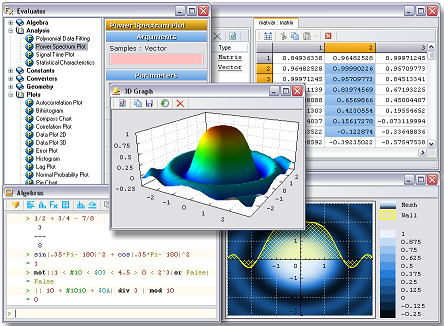## Research & DevelopmentsAlgebrus software along with the specially developed XPascal scientific programming language is rich on computational capabilities and has plenty of features that make any kind of calculations fast and easy. It could be as powerful as an expensive software for mathematical calculations or as simple as small "evaluate-while-type" expression calculator (in the compact console mode). And now it is FREE!

Important: under Windows 8 or higher the software needs to be launched "as administrator".

XPascal programming language

• Native support for vectors and matrices
• User-defined operators for user-defined types
• Structured user-defined types
• Support for interactive constant routine parameters
• Chained assignment and relational expressions
• Dynamic and static arrays
• Efficient array access loop statements
• Rich on high level routines for scientific analysis
• Easy to learn simplified yet powerful grammar

Calculations

• Operations with vectors, matrices, polynomials, and fractions
• Complex and quaternion numbers support
• More than 250 built-in mathematical and analysis routines
• User-programmed procedures and functions in XPascal language
• Local and global multi-variable optimisation
• Algebraic and differential equations and equation system solving
• Linear algebra, eigen values, Fourier analysis and many more
• Geometry problems solving
• Statistical data analysis and data fitting
• Converting to and from many dimensions of 20 different kinds of physical and mathematical quantities
• More than 100 fundamental physical constants

Graphs plotting

• 2D and 3D graphic plots of functions and data
• Lines, parametric lines, surfaces, height maps, contour lines
• Scattered plots, error and field plots, different charts and diagrams
• Spectrum, Histogram, bihistogram, probability, lag, correlation plots
• Compass and pie charts
• Linear, semi-log, logarithmic scales and polar coordinate system
• Highly customisable graphs appearance and realistic rendering
• Plotting graphs in many different but simple ways
• Exporting graphics as bitmaps and metafiles
• Interactive arbitrary rotation of 3D graphs

Data import and export

• Import and export data from and to standard and custom formats
• Files, clipboard, and RS-232 as data sources are supported
• User programmable type specific data import/export filters
• Copy/Paste operations with standard formats
• Variable of any type (including user-defined) can be serialised by a single function call

User interface

• Simple and interactive user interface for functions evaluation
• Compact and full console modes
• "Evaluate-while-typing" mode
• Decimal, binary, and hexadecimal integer formats
• Syntax-highlighted XPascal editor
• Syntax-highlighted command console and expression editors
• Context based help search
• Highly customizable user interface